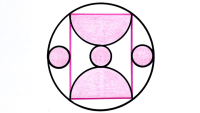# Catriona Agg’s Puzzles from 2021

1. three squares ii May 2, 2021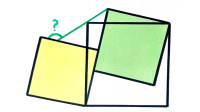2. two overlapping triangles ii Apr 11, 2021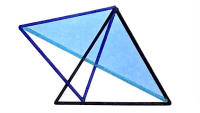3. two regular hexagons iii Apr 10, 2021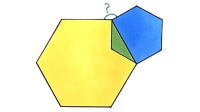4. isosceles and equilateral triangles Apr 10, 2021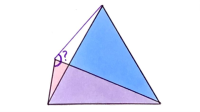5. two concentric circles Apr 10, 2021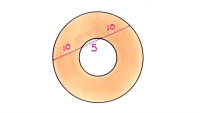6. four squares and a quarter circle Apr 9, 2021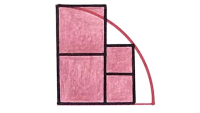7. hexagon and a square Apr 6, 2021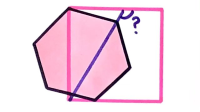8. two quarter circles in a square ii Apr 5, 2021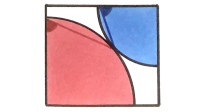9. four squares in a semi-circle Apr 4, 2021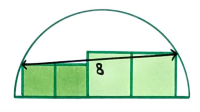10. triangle in two tilted squares Apr 3, 2021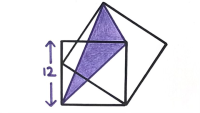11. quarter circle overlapping a circle Apr 2, 2021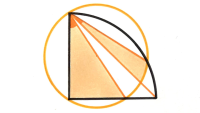12. five equilateral triangles Apr 1, 2021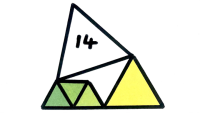13. four equilateral triangles inside a hexagon Mar 31, 2021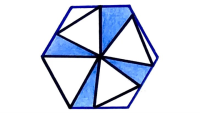14. two quarter circles in a semi-circle ii Mar 30, 2021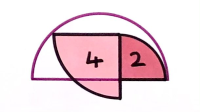15. three squares iii Mar 29, 2021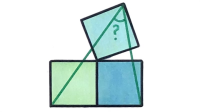16. four squares viii Mar 28, 2021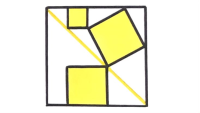17. seven quarter circles inside a semi-circle Mar 27, 2021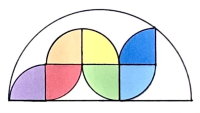18. two hexagons and a triangle Mar 26, 2021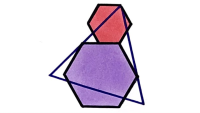19. two squares inside a semi-circle Mar 23, 2021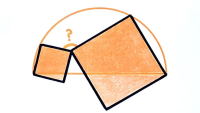20. five semi-circles Mar 22, 2021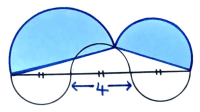21. four squares vii Mar 21, 2021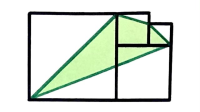22. four parallel semi-circles Mar 20, 2021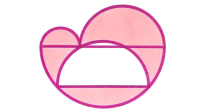23. three overlapping hexagons Mar 19, 2021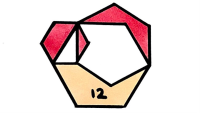24. four squares vi Mar 18, 2021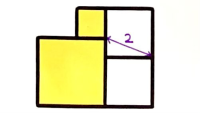25. two squares on a hexagon Mar 17, 2021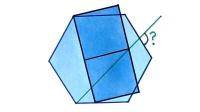26. crossed rectangles in a square Mar 16, 2021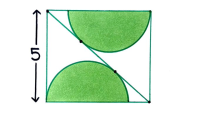27. three triangles in a hexagon Mar 15, 2021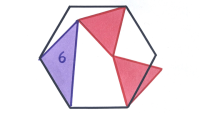28. striped semi-circle Mar 14, 2021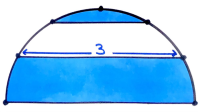29. two quarter circles and a semi-circle ii Mar 13, 2021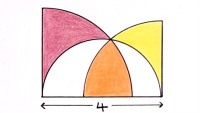30. five quarter circles Mar 12, 2021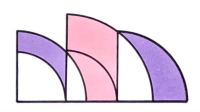31. subdivided hexagon Mar 10, 2021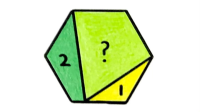32. multiple semi-circles iii Mar 7, 2021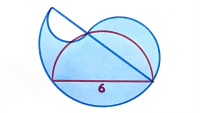33. three overlapping semi-circles Mar 6, 2021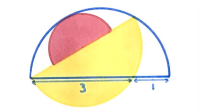34. three quarter circles Mar 2, 2021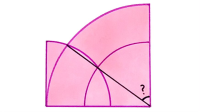35. two squares on a diameter of a circle Mar 1, 2021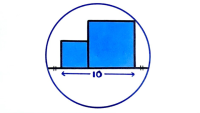36. a hexagon and a nonagon Feb 28, 2021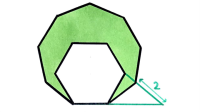37. four quarter circles in a circle Feb 27, 2021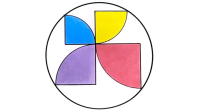38. three regular hexagons iii Feb 26, 2021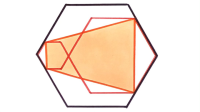39. two squares overlapping a square ii Feb 23, 2021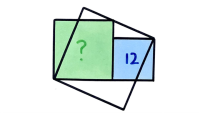40. three congruent triangles ii Feb 21, 2021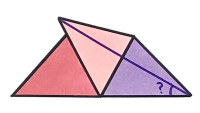41. two triangles overlapping a quarter circle Feb 20, 2021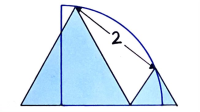42. three congruent rectangles iv Feb 19, 2021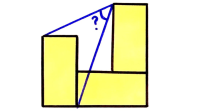43. three congruent triangles in a semi-circle Feb 18, 2021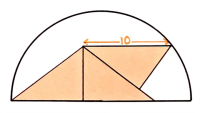44. triangle over a circle Feb 17, 2021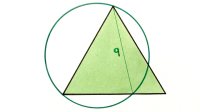45. multiple semi-circles iv Feb 16, 2021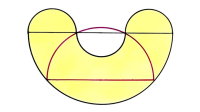46. four squares v Feb 15, 2021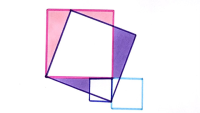47. two squares overlapping a square Feb 14, 2021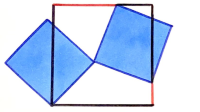48. zig-zag inside a square Feb 13, 2021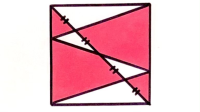49. two semi-circles in a semi-circle Feb 11, 2021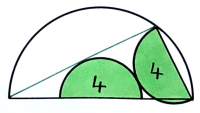50. six triangles in a hexagon Feb 9, 2021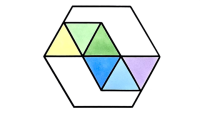51. two overlapping triangles Feb 7, 2021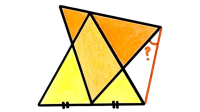52. four squares inside a rectangle Jan 31, 2021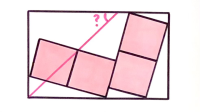53. two triangles in a circle and semi-circle Jan 30, 2021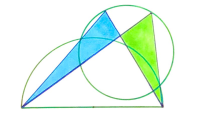54. triangle in a rectangle Jan 29, 2021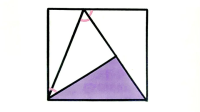55. multiple semi-circles ii Jan 27, 2021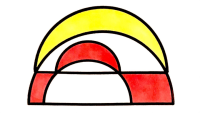56. square overlapping a square and a rectangle Jan 24, 2021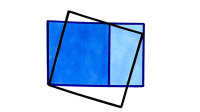57. chord splitting a square in half Jan 23, 2021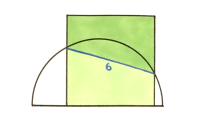58. rectangle tilted over a square Jan 16, 2021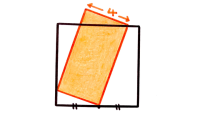59. multiple angles Jan 10, 2021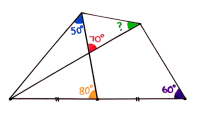60.